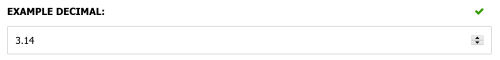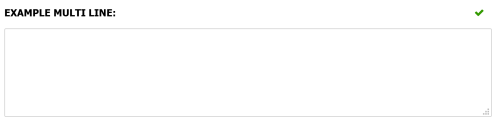# Primitives

Primitive and basic types such as `string` and `int` display using a default editor that corresponds with their type. The default editor can be overridden by adding data annotations.

Supported primitive types include:

• `bool`: Displays as a checkbox
• `char`: Displays as a single-character text input
• `string`: Displays as a text input
• `byte`, `sbyte`, `short`, `ushort`, `int`, `uint`, `long`, `ulong`: Displays as an integer number input
• `float`, `double`, `decimal`: Displays as a numerical input

The following data annotations can be used to enhance the standard editors:

Each of these are explained below:

## [Number]

The `[Number]` data annotation can be used to decorate a numeric field (e.g. int, decimal, long) and provide a UI hint to the admin interface to display an html5 number field.

The step property can be used to specify the precision of the number e.g. 2 decimal places

A nullable numeric type indicates it is an optional field, while a non-null numeric type indicates it is a required field.

#### Optional parameters

• Step: Maps to the step attribute on a number html input field. This can be used to control the precision of the number entered. E.g. use a step value of '0.1' to allow a decimal value to 1 decimal place. The default value is '1' which uses integer level precision. A special value of 'any' can be used to allow any number type.

#### Example

``````public class ExampleDataModel : ICustomEntityDataModel
{
/// <summary>
/// Renders a numeric input to two decimal places. As the decimal
/// type is nullable, this is an optional field.
/// </summary>
[Number(Step = "0.01")]
public decimal? ExampleDecimal { get; set; }
}
``````

Output:## [MultiLineText]

The `[MultiLineText]` data annotation can be used to decorate a `string` property and provide a UI hint to the admin interface to display a textarea field.

#### Optional parameters

• Rows: The number of visible lines of text in the text editor. Defaults to 4.

#### Example

``````public class ExampleDataModel : ICustomEntityDataModel
{
[MultiLineText(Rows = 8)]
public string ExampleMultiLine { get; set; }
}
``````

Output: